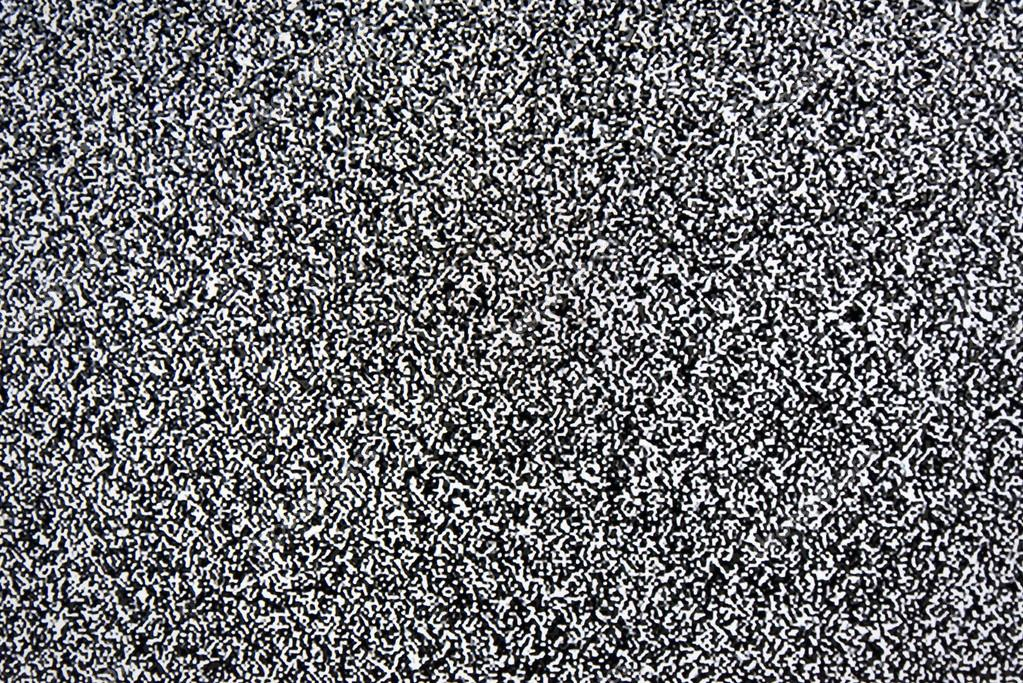# Step to the neutron stars 10. Noise#### A step back to the radiometer equation

There some points which bother me and I think it is important to mention. The RMS value of noise power (thermal noise) $P$ in the bandwidth of $B$ is:

$P=k_BT_{sys}B$

where $T_{sys}$ is system temperature, and $k_B$ Boltzman constant. So, the noise of the system can be described by the value of temperature. In the period of time $\tau$ we make $N=2B\tau$ independent measures of the noise power. Each of it have RMS deviation $\sigma_T=\sqrt{2}T_{sys}$. The square root appears here because of variable change from field to power (in detail here). We need to make this change because $T_{sys}$ determines the RMS in amplitude. To get the RMS in power, we use central limit theorem:

$\sigma_P=\frac{\sigma_T}{\sqrt{N}}$

According to Nyqust theorem:

$\sigma_T=\frac{\sqrt{2}T_{sys}}{\sqrt{2B\tau}}=\frac{T_{sys}}{\sqrt{B\tau}}$

And so we have radiometer equation.

#### Noise contributions

The system temperature $T_{sys}$ have several contibutions:

1. $T_{rec}$ -- receiver noise (20 K).
2. $T_{spill}$ -- antenna noise: throw sidelobes (from the earth), RFI and by itself (10 K).
3. $T_{sky}$ -- the noise of atmosphere and of the sky (10-30 K).

If the noise of the sky I cannot change, so the noise of the electronics I can improve. The most critical in this sense is the noise of the first amplifier after the antenna. Each amplifier has its noise level or "noise floor".

#### Amplifier noise

The amplifier noise is characterized by the quantity called Noise Figure:

$NF=10\times log_{10}(F)$

where F -- is a factor of noise. By definition, it is a ratio of the total output power to the power of the input source noise. For the amplifier with low noise (low noise amplifier -- LNA), NF doesn't exceed 1 dB.

There are three main methods to measure the noise floor:

#### Gain method

This is one of the most popular methods. The input of the amplifier is shortened by 50 Ohm resistor. As was mentioned above, the thermal power noise of this source is $P=k_BTB$. At $290 K$ we have $k_BT=-174 dBm/Hz$, so:

$NF=P_{Nout}-(-174 dBm/Hz+10log_{10}(BW)+Gain)$

where $P_{Nout}$ is measured power at the output of the amplifier. One of the disadvantages of this method is the necessity of independent measurements of the bandwidth and gain of this amplifier.

However, this method doesn't work well for the LNA as it requires a very precise power meter.

#### Y factor method

In this case, the input of the amplifier is connected to a noise source with certain ENR (Excess Noise Ratio in dB) and on the output, we measure the difference (in dB) in cases when the noise source is on and off (it is a Y factor). Then the noise figure is:

$NF=10log_{10}\frac{10^{ENR/10}}{10^{Y/10}-1}$

The third method is measurements with a specialized device, but it is also based on the Y factor method.

The noise factor can be converted to temperature:

$T=T_{ref}\times (F-1)$

as $T_{ref}$ is taken 290 К. Conversion Noise Figure - the temperature can be done here or using a table at the left.

Step to the neutron stars 10. Noise
Scroll to top